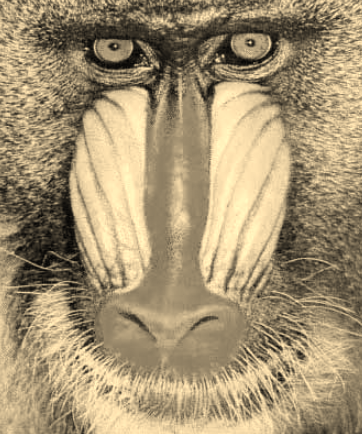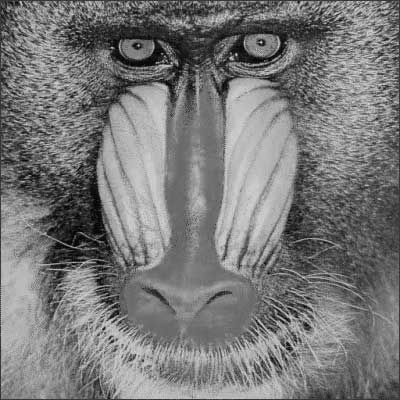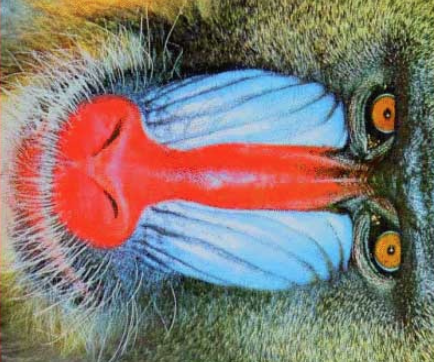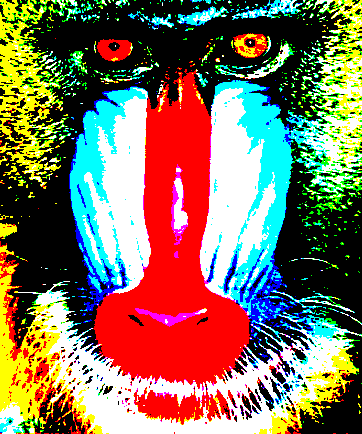## Class 36 — November 26

#### Image visualization

I draw from the past – Wonder about the present – Think of the future

### Sepia

• There is no one formula for defining a Sepia pixel conversation. The one used here comes from Microsoft.
• For pixel `(r,g,b)`, the Sepia conversion is `(sr,sg,sb)` where

sr, sg, sb = int( min( i, 255 )), int( min( j, 255 )), int( min( k, 255 )),

where `i, j, k` are

i = .393r + .769g + .189b

j = .349r + .686g + .168b

k = .272r + .534g + .131b

### Palette reduction

• Sometimes to save computer memory, software developers perform a palette reduction of colors used in image representation.
• Our reduction scheme will use an eight-color palette
• Black, White, Red, Green, Blue, Yellow, Magenta, and Cyan; that is, `(0,0,0), (255,255,255), (255,0,0), (0,255,0), (0,0,255), (255,255,0), (255,0,255),` and `(0,255,255)`
• In a palette reduction, a pixel `(r,g,b)` is replaced by the pixel in in the palette it is closest to. The distance between pixels `(r1,g1,b1)` and `(r2,g2,b2)` equals

abs(r1 - r2) + abs(g1 - g2) + abs(b1 - b2)